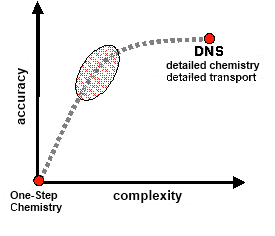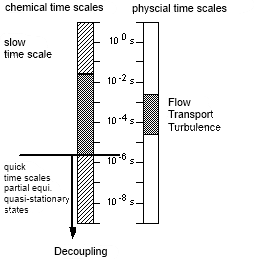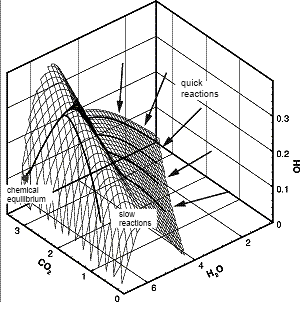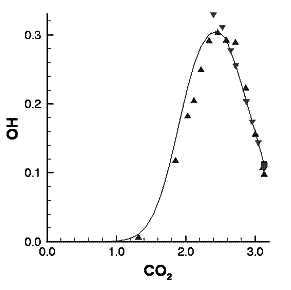# Hierarchical modelling concepts

In order to solve the aforementioned problems associated with DNS, modelling assumptions need to be made for the chemical reaction, the turbulent flow field and the multiphase processes. Unfortunately, the models normally used nowadays in commercial simulation programs mostly oversimplify the complex processes, for example in the interaction of chemical reactions with the turbulence. Each decrease in complexity during modelling involves a certain loss of accuracy, as shown schematically on the left in Fig. 4.

The objective is to achieve substantial model reduction with a minimal loss of accuracy. The question therefore arises, whether the information from detailed simulations could be used for improving the modelling assumptions. We will show below how a hierarchical procedure can be used to solve this problem. For example,  detailed experiments and detailed simulations allow reduced models to be derived, which can then be used as sub-models in the description of technical combustion systems. In this way, information about the processes, at the smallest time and distance scales, is incorporated into the global models.

#### Reduction of the chemical kinetics

For reasons of computing time, usually simulations of technical combustion processes rely on reduced reaction mechanisms. Such reduced reaction mechanisms describe the chemical kinetics as a function of just a few sp-called reaction progress variables, exploiting systematically the fact that rapid chemical processes lead to correlations of the species concentrations in state space.

The reason for this is the wide range of time-scales in the reaction system. Whereas the chemical processes vary over time-scales in the range of approx. 10 orders of magnitude, the range of physical time-scales (time-scales of turbulence and molecular transport) is very much smaller (see Fig. 4 on the right). If we consider the dynamics of the chemical reaction, we find that rapid chemical processes lead to relaxation of the thermokinetic states to low-dimensional manifolds. This is shown in Fig. 5. Several trajectories of the chemical kinetics are plotted for different initial conditions, which after a very short time relax to a two-dimensional surface, then to a one-dimensional curve and finally to the equilibrium point.Graph showing the accuracy attainable in simulations of combustion processes as a function of the complexity of the underlying models. The complexity need not be increased very much in order to come very close to the maximum accuracy (DNS). The current development of new simplified models takes place approximately within the shaded ellipse. Typical time-scales of combustion processes.

One of the methods used at the Institute of Technical Thermodynamics for identifying low-dimensional manifolds and thus for computer-aided reduction of reaction mechanisms, is the method of intrinsic low-dimensional manifolds (ILDM) shown schematically in Fig. 5.Reduction of large reaction mechanisms by means of the ILDM method, using as an example the combustion of a methane/air mixture. Rapid chemical processes are identified and regarded as quasi-stationary. Verification of the reduced computation is possible through comparison with a detailed computation. Curve: detailed mechanism, symbols: reduced mechanism with three reaction progress variables. Each shows the ratio of the species mass to the total mass in mol/kg.

This method identifies rapid chemical processes that lead to local quasi-stationary states, and decouples them so that the system of species conservation equations can be reduced to a very small number of reaction progress variables. Using the ILDM method, even very complex chemical reaction systems can be described with a small number of conservation equations. For example in the combustion of dodecane, in which ca. 1000 chemical species are involved in the reaction, in the high-temperature range even a reduced system with 2 to 3 progress variables allows a sufficiently precise description of the reaction process.

Start | Previous | Page: 1 - 2 - 3 - 4 - 5 - 6 | Next | End